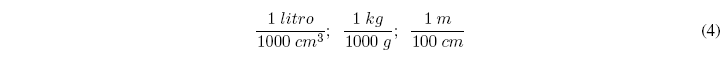The conversion factors are based on multiplying by fractions that have the same quantity in the numerator and denominator but in different units. Some examples of unit factors are the following:In the conversion factors, the units guide us in the calculations. All the units are canceled until reaching the desired result.

Let's see an application of the conversion factors: Knowing that one erg is equal to 1 10 -7 joules. Convert 3.74 10 -2 ergs to joules.

The solution can be obtained by applying conversion factors: